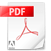# Taking Bearings on a Nautical Chart

Overview:

A = Make sure the arrow is pointing in the direction you wish to travel

B = Make sure the arrows are pointing to North on the Chart

C = Read off the bearing

Calculating effective bearings involves:

• T - True Course in Degrees,
• V - Apply Variation,
• M - to give us a Magnetic course,
• D - Apply Variation,
• C - to give us a Compass Course.

## Example:

1) PICK TWO POINTS YOU WISH TO TRAVEL BETWEEN ON THE CHART (Ensure there aren't any hazards between!)

2) LINE UP THE PLOTTER BETWEEN THE FIRST AND SECOND POINT, MAKING SURE THE DIRECTION OF BEARING ARROW ON YOUR PLOTTER IS POINTING IN THE DIRECTION YOU WISH TO TRAVEL.

3) LINE UP THE COMPASS ON THE PLOTTER WITH THE GRID LINES ON THE CHART

4) TURN THE PLOTTERS COMPASS UNTIL THE 'N' IS POINTING TO NORTH ON THE CHART

5) THE NUMBER ON THE PLOTTERS COMPASS BELOW THE '0' IS YOUR ‘TRUE’ BEARING. IN THIS EXAMPLE IT IS 291 DEGREES (TRUE)

## Now we need to consider Variation:

WHAT IS VARIATION?

Variation is the difference between True and Magnetic North.

The Longitude Lines on our Chart point to the 'True' North Pole

Our compass points to the 'Magnetic' North Pole.

Variation varies dependant on where you are in the world so always check Variation on the Compass Rose on an up to date local chart.

There are plenty of mnemonic's to remember how to calculate Variation. We think the simplest is 'Easy is Least, West is Best'

I.E. when the Variation is East you SUBTRACT it from your True Bearing in order to gain your Magnetic Bearing. ; if the Variation is West you ADD it to your True Bearing  in order to gain your Magnetic Bearing.

6) CALCULATE VARIATION: LOCATE THE COMPASS ROSE ON YOUR CHART NEAREST TO YOUR POINT OF TRAVEL (BEARING)

7) IN THIS EXAMPLE: THE VARIATION MARKED ON THE CHART IS 2 DEGREES 35’ WEST.

8) TO CALCULATE VARIATION USE THE FOLLOWING:

IN THIS EXAMPLE:

TRUE BEARING  = 291 DEGREES

VARIATION = 2 DEGREES WEST (ACCURACY IS ONLY NEED TO BE TO THE NEAREST DEGREE FOR SHORTER DISTANCES)

THEREFORE = 291 (T) + 2 (V) = 289 DEGREES (M) WHICH IS THE MAGNETIC BEARING

AS ITS NEARLY IMPOSSIBLE TO STEER TO 'A DEGREE' WHEN USING THIS AFLOAT (ASSUMING YOU'RE TRAVELLING RELATIVELY SHORT DISTANCES ROUND UP OR DOWN TO THE NEAREST '0' OR '5' TO CREATE A 'USABLE' BEARING, SO IN THIS CASE 290 DEGREES (M)

## And now another consideration, Deviation:

WHAT IS DEVIATION?

Compass Deviation is caused by the magnetic fields generated onboard your boat i.e. engines, electronics, metal work etc. Each vessel will have its own unique deviation which is calculated and detailed on a 'deviation card'.

Once Deviation is known we revert back to the mnemonic at the beginning of this page:

• T - 'True Bearing'
• V - Apply Variation
• M - Gives us a 'Magnetic Bearing'
• D - Apply Deviation
• C - to give us a 'Compass Bearing'

This is also where the mnemonic CADET effectively comes into play.

Example of a Deviation card:Deviation Card Template.pdf

# F.A.Q.s.

What is a True North?

True North is where the Longitude Lines meet at the North Pole.

What is Variation?

In a nutshell it is the difference between True North and Magnetic North.

True North is where the Longitude Lines meet at the North Pole. Magnetic North is where our compass points which is towards the Magnetic North Pole; how much variation and whether it lies to the East or West depends on where we are in the world. Variation is shown on the Compass Rose on your Chart.

What is Compass Deviation?

Deviation is created by items such as electronics, metal work, engines etc on your boat all of which create their own magnetic field which in-turn effects your compass. Every vessel will therefore have its own unique variation which can be calculated and logged on a Deviation Table.